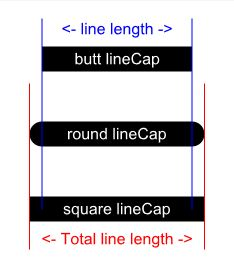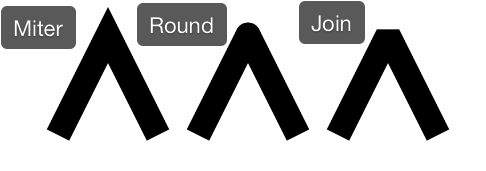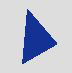Class

# WebGraphics

## Description

WebGraphics class objects are used for drawing text, lines, rectangles, ovals, and pictures from within the a WebCanvas event.

## Methods

Name

Parameters

Returns

Shared

ClearRectangle

X As Integer, Y As Integer, Width As Integer, Height As Integer

DrawLine

X1 As Integer, Y1 As Integer, X2 As Integer, Y2 As Integer

DrawOval

X As Integer, Y As Integer, Width As Integer, Height As Integer

DrawPicture

p As Picture, x As Integer, y As Integer

DrawPolygon

Points() As Integer

DrawRectangle

X As Integer, Y As Integer, Width As Integer, Height As Integer

DrawRoundRectangle

x As Integer, y As Integer, width As Integer, height As Integer, ArcWidth As Integer

DrawText

text As String, x As Integer, y As Integer, [width As Integer]

FillOval

X As Integer, Y As Integer, Width As Integer, Height As Integer

FillPolygon

Points() As Integer

FillRectangle

X As Integer, Y As Integer, Width As Integer, Height As Integer

FillRoundRectangle

x As Integer, y As Integer, width As Integer, height As Integer, ArcWidth As Integer

Restore

Save

Size As Integer, Col As Color, OffsetX As Integer, OffsetY As Integer

## Enumerations

WebGraphics.LineCapTypes

### LineCapTypes

Specifies the types of caps (endings) that will be drawn on the end of lines drawn with the DrawLine method.

Enum

Description

Butt

A squared-off ending that ends at the line boundary.

Round

A rounded ending that extends beyond the line boundary.

Square

A squared-off ending that extends beyond the line boundary.

WebGraphics.LineJoinTypes

### LineJoinTypes

Specifies the type of path to be used to open the folderitem.

Enum

Description

Butt

A squared-off ending that ends at the line boundary.

Round

A rounded ending that extends beyond the line boundary.

Square

A squared-off ending that extends beyond the line boundary.

## Property descriptions

WebGraphics.Bold

Bold As Boolean

If True, applies the bold style to text drawn using WebGraphics.

In the WebCanvas event handler, this code displays bold text:

```g.Bold = True
g.DrawText("Hello World!", 10, 100)
```

WebGraphics.DrawingColor

DrawingColor As Color

The currently selected color for the WebGraphics object. This color is used by the various drawing methods.

This example draws a red rectangle:

```g.DrawingColor = Color.Red
g.FillRectangle(10, 10, 100, 100)
```

WebGraphics.FontName

FontName As String

Name of the font used to draw text with the DrawText method.

You can use any font supported by the web browser. For best results you will want to stick with the standard Web Safe Fonts.

```g.FontName = "Arial"
g.DrawText("Hello World!", 10, 10)
```

WebGraphics.FontSize

FontSize As Integer

Sets the size of the text to display using the DrawText method.

This code draws large text:

```g.FontSize = 48
g.DrawText("Hello World!", 10, 100)
```

WebGraphics.Height

Height As Integer

The height in pixels of the drawing areas of the parent WebCanvas.

This example gets the height of the parent WebCanvas and draws a line down the left edge:

```Var h As Integer
h = g.Height
g.PenSize = 5
g.DrawLine(0, 0, 0, h)
```

WebGraphics.Italic

Italic As Boolean

If True, text will appear in italic when using WebGraphics.

This example draws "Hello World" in red italic inside a WebCanvas.

```g.DrawingColor = &cff0000
g.Italic = True
g.DrawText("Hello world", 10, 10)
```

WebGraphics.LineCap

LineCap As LineCapTypes

Specified Butt, Round or Square as the Line Cap.

Use WebGraphics.LineCapType enumeration to set the LineCap. Values are Butt, Round and Square.```g.LineCap = WebGraphics.LineCapType.Round
```

WebGraphics.LineJoin

LineJoin As LineJoinTypes

Specified Bevel, Miter, Round as the Line Join.

Use WebGraphics.LineJoinType enumeration to set the LineJoin. Values are Bevel, Miter and Round.```g.LineJoin = WebGraphics.LineJoinType.Miter
```

WebGraphics.PenSize

PenSize As Integer

The drawing pen is always square, so this specifies both the width/height (in pixels) used when drawing lines, ovals and rectangles.

This example sets the PenSize to 5 pixels to draw a rectangle with a thick border:

```g.PenSize = 5
g.DrawRectangle(150, 10, 100, 100)
```

WebGraphics.TextAlignment

TextAlignment As TextAlignments

Sets the text alignment of text created with DrawText.

This code centers the text in the drawing area:

```Var txt As String = "Hello World"
Var y As Integer = 100
Var alignX As Integer = g.Width / 2
g.TextAlignment = TextAlignments.Center
g.DrawText(txt, alignX, y)
```

Show left-aligned, right-aligned and centered text, both within a Rect and within the entire Canvas. Be sue the Canvas is locked to all page edges so that it is large enough:

```g.DrawRectangle(0, 10, 200, 100)

g.TextAlignment = TextAlignments.Left
g.DrawText("Left-aligned (Box)", 5, 20) ' in box
g.DrawText("Left-aligned (Canvas)", 5, 200, g.Width) ' in canvas

g.TextAlignment = TextAlignments.Right
g.DrawText("Right-aligned (Box)", 195, 40) ' in box
g.DrawText("Right-aligned (Canvas)", g.Width, 250, g.Width) ' in canvas

g.TextAlignment = TextAlignments.Center
g.DrawText("Centered (Box)", 100, 60) ' in box
g.DrawText("Centered (Canvas)", g.Width / 2, 300, g.Width) ' in canvas
```

This code splits a number into two parts so you can do decimal alignment:

```Var c As Currency = 15.32
Var cText As String = c.ToString
Var parts() As String = cText.ToArray(".")
Var y As Integer = 100
Var alignX As Integer = g.Width/2
g.TextAlignment = WebGraphics.TextAlignmentRight
g.DrawText(parts(0) + ".", alignX, y)
g.TextAlignment = WebGraphics.TextAlignmentLeft
g.DrawText(parts(1), alignX, y)
```

WebGraphics.Width

Width As Integer

The width in pixels of the drawing areas of the parent WebCanvas.

This example gets the width of the parent WebCanvas and draws a line from the top left to top right:

```Var w As Integer
w = g.Width
g.PenSize = 5
g.DrawLine(0, 0, w, 0)
```

## Method descriptions

WebGraphics.ClearRectangle

ClearRectangle(X As Integer, Y As Integer, Width As Integer, Height As Integer)

Clears the rectangle described by the parameters passed by filling it with the background color.

This example clears the entire drawing area:

```g.ClearRectangle(0, 0, g.Width, g.Height)
```

WebGraphics.DrawLine

DrawLine(X1 As Integer, Y1 As Integer, X2 As Integer, Y2 As Integer)

Draws a line from x1, y1 to x2, y2 in the current color (specified by ForeColor).

Draws a line from the top left to the bottom right:

```g.PenSize = 5
g.DrawLine(0, 0, g.Width, g.Height)
```

WebGraphics.DrawOval

DrawOval(X As Integer, Y As Integer, Width As Integer, Height As Integer)

Draws the outline of an oval in the current color (specified by ForeColor property). x and y are the coordinates of the top-left corner. width and height specify the size of the oval.

The following example sets the width and draws the oval. The code is in the Paint event of a WebCanvas.

```g.PenSize = 5
g.DrawOval(10, 10, 100, 60)
```

WebGraphics.DrawPicture

DrawPicture(p As Picture, x As Integer, y As Integer)

Draws the picture from a WebImageViewer at the specified location. The picture can be shown at full size or cropped. All units are pixels.

x and y are the distances from the top-left corner of the control or window from which the 0,0 point of the image will be drawn. The optional parameters are used to copy a portion of the picture for cropping.

If you are cropping, then width and height are required.

The following code crops the image. It copies only the top 150 pixels of the original image at full size:

```g.DrawPicture(myImage, 0, 0, 600, 150)
```

This draws the full image:

```g.DrawPicture(myImage, 0, 0)
```

WebGraphics.DrawPolygon

DrawPolygon(Points() As Integer)

Draws a polygon using the values in the 1-based array passed as the X and Y coordinates. The polygon is drawn in the current color (specified by ForeColor). Odd numbered array elements are X coordinates and even numbered array elements are Y coordinates.

Polygons are drawn using the DrawPolygon and FillPolygon methods of the WebGraphics class. Polygons are drawn by passing the DrawPolygon or FillPolygon method an Integer array that contains each point in the polygon. This is a 1-based array where odd numbered array elements contain X values and even numbered array elements contain Y coordinates. This means that element 1 contains the X coordinate of the first point in the polygon and element 2 contains the Y coordinate of the first point in the polygon. Consider the following array values:

Element #

Value

1

10

2

5

3

40

4

40

5

5

6

60

When passed to the DrawPolygon or FillPolygon method, this array would draw a polygon by drawing a line starting at 10,5 and ending at 40,40 then drawing another line starting from 40,40 ending at 5,60 and finally a line from 5,60 back to 10,5 to complete the polygon. This polygon has only three sets of coordinates so it is a triangle.

This code is in the Paint event of a WebCanvas control:

```Var points(6) As Integer
points(1) = 10
points(2) = 5
points(3) = 40
points(4) = 40
points(5) = 5
points(6) = 60
g.DrawPolygon(points)
```

FillPolygon draws the same polygon but with the interior filled with the ForeColor:Another way of populating the array is with the Array function. The Array function takes a list of values separated by commas and populates the array, beginning with element zero. Since the first element that DrawPolygon uses is element 1, you can use any value in element zero. This code is in the Paint event.

```Var points() As Integer
points = Array(0, 10, 5, 40, 40, 5, 60)
g.DrawPolygon(points)
```

WebGraphics.DrawRectangle

DrawRectangle(X As Integer, Y As Integer, Width As Integer, Height As Integer)

Draws the outline of a rectangle in the DrawingColor. x and y are the coordinates of the top-left corner. width and height specify the size of the rectangle.

This example draws two squares with a 5-pixel red border:

```g.DrawingColor = Color.Red
g.PenSize = 5
g.DrawRectangle(10, 10, 100, 100)
g.DrawRectangle(130, 10, 100, 100)
```

WebGraphics.DrawRoundRectangle

DrawRoundRectangle(x As Integer, y As Integer, width As Integer, height As Integer, ArcWidth As Integer)

{

x and y are the coordinates of the top-left corner. width and height specify the size of the round rectangle. ArcWidth controls the shape of the corners of both the horizontal and vertical axes. It is the distance (in pixels) from the corner at which the arc begins. Setting ArcWidth to zero results in a rectangle with sharp corners (a normal rectangle).}}

This example draws two squares with a 5-pixel red border. The left square sets the arcs to 10 and the right one to 25.

```g.DrawingColor = Color.Red
g.PenSize = 5
g.DrawRoundRectangle(10, 10, 100, 100, 10)
g.DrawRoundRectangle(130, 10, 100, 100, 25)
```

WebGraphics.DrawText

DrawText(text As String, x As Integer, y As Integer, [width As Integer])

Draws the text at the specified location and in the DrawingColor.

The x parameter specifies the distance from the left of the WebGraphics object in pixels. The y parameter specifies the baseline for the text. The optional width parameter specifies the width (in pixels) at which text should wrap. If width is omitted, then text will print on one line, even if the drawing area is too narrow to contain the text.

This example draws text in red Helvetica 16-point.

```g.DrawingColor = Color.Red
g.FontName = "Helvetica"
g.FontSize = 16
g.DrawText("Hello world!", 10, 130)
```

WebGraphics.FillOval

FillOval(X As Integer, Y As Integer, Width As Integer, Height As Integer)

Draws an oval filled with the current color (specified by ForeColor). x and y are the coordinates of the top-left corner. width and height specify the size of the oval.

The following example draws a filled oval. The code is in the Paint event of a WebCanvas:

```g.DrawingColor = &cff0000
g.FillOval(10, 10, 100, 60)
```

WebGraphics.FillPolygon

FillPolygon(Points() As Integer)

Draws a polygon using the values in the 1-based array passed as the X and Y coordinates. The polygon is filled using the DrawingColor. Odd numbered array elements are X coordinates and even numbered array elements are Y coordinates.

Polygons are drawn using the DrawPolygon and FillPolygon methods of the WebGraphics class. Polygons are drawn by passing the DrawPolygon or FillPolygon method an Integer array that contains each point in the polygon. This is a 1-based array where odd numbered array elements contain X values and even numbered array elements contain Y coordinates. This means that element 1 contains the X coordinate of the first point in the polygon and element 2 contains the Y coordinate of the first point in the polygon. Consider the following array values:

Element #

Value

1

10

2

5

3

40

4

40

5

5

6

60

When passed to the DrawPolygon or FillPolygon method, this array would draw a polygon by drawing a line starting at 10,5 and ending at 40,40 then drawing another line starting from 40,40 ending at 5,60 and finally a line from 5,60 back to 10,5 to complete the polygon. This polygon has only three sets of coordinates so it is a triangle.

This code is in the Paint event of a WebCanvas control:

```Var points(6) As Integer
points(1) = 10
points(2) = 5
points(3) = 40
points(4) = 40
points(5) = 5
points(6) = 60
g.FillPolygon(points)
```

FillPolygon draws the same polygon but with the interior filled with the ForeColor:Another way of populating the array is with the Array function. The Array function takes a list of values separated by commas and populates the array, beginning with element zero. Since the first element that DrawPolygon uses is element 1, you can use any value in element zero. This code is in the Paint event.

```Var points() As Integer
points = Array(0, 10, 5, 40, 40, 5, 60)
g.FillPolygon(points)
```

WebGraphics.FillRectangle

FillRectangle(X As Integer, Y As Integer, Width As Integer, Height As Integer)

{

x and y are the coordinates of the top-left corner. width and height specify the size of the rectangle.}}

This example draws two red squares:

```g.DrawingColor = Color.Red
g.FillRectangle(10, 10, 100, 100)
g.FillRectangle(130, 10, 100, 100)
```

WebGraphics.FillRoundRectangle

FillRoundRectangle(x As Integer, y As Integer, width As Integer, height As Integer, ArcWidth As Integer)

{

x and y are the coordinates of the top-left corner. width and height specify the size of the round rectangle. ArcWidth controls the shape of the corners of both the horizontal and vertical axes. It is the distance (in pixels) from the corner at which the arc begins. Setting ArcWidth to zero results in a rectangle with sharp corners (a normal rectangle). }}

This example draws two red rounded squares. The left square sets the arcs to 10 and the right one to 25.

```g.DrawingColor = Color.Red
g.FillRoundRectangle(10, 10, 100, 100, 10)
g.FillRoundRectangle(130, 10, 100, 100, 25)
```

WebGraphics.Restore

Restore

Pops the top state on the stack, restoring the graphics context to that state.

WebGraphics.Save

Save

Saves the current state of the graphics context onto the stack.

Shadow(Size As Integer, Col As Color, OffsetX As Integer, OffsetY As Integer)

Renders shadows for all drawing commands that follow * Size: How much to blur the shadow * Col: Color of the shadow * OffsetX: How far to move the shadow to the right * OffsetY: How far to move the shadow downward

Draws a blue rectangle with a red shadow:

```g.Shadow(12, &cff0000, 3, 3)
g.DrawingColor = &c0000ff
g.FillRectangle(10, 10, 100, 100)
```

## Compatibility

Web projects on all supported operating systems.